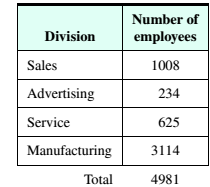# Computer Allocation The following table shows the number of employees in the four divisions of a corporation. There are 85 new computers that are to be apportioned among the divisions based on the number of employees in each division. a. Lice the Hamilton method to determine the number of computers to be apportioned to each division. b. Use the Jefferson method to determine the number of computers to be apportioned o each division. Does this particular apportionment violate the quota rule?### Mathematical Excursions (MindTap C...

4th Edition
Richard N. Aufmann + 3 others
Publisher: Cengage Learning
ISBN: 9781305965584

#### Solutions

Chapter
Section### Mathematical Excursions (MindTap C...

4th Edition
Richard N. Aufmann + 3 others
Publisher: Cengage Learning
ISBN: 9781305965584
Chapter 4, Problem 2T
Textbook Problem
2 views

## Computer Allocation The following table shows the number of employees in the four divisions of a corporation. There are 85 new computers that are to be apportioned among the divisions based on the number of employees in each division.a. Lice the Hamilton method to determine the number of computers to be apportioned to each division.b. Use the Jefferson method to determine the number of computers to be apportioned o each division. Does this particular apportionment violate the quota rule?

To determine

(a)

Use the Hamilton method to determine the number of computers to be apportioned to each division.

### Explanation of Solution

Given Information:

 Divisions Number of Employees Quotient Standard Quota Number of Computers Sales 1008 100858.6≈17.201 17 17 Advertising 234 23458.6≈3.993 3 4 Service 625 62558.6≈10.665 10 11 Manufacturing 3114 311458.6≈53.139 53 53 Total 83 85

Concept used:

The Hamilton/Vinton Method

The Hamilton/Vinton Method sets the divisor as the proportion of the total population per house seat. After each states population is divided by the divisor the whole number of the quotient is kept and the fraction dropped.

This will result in surplus house seats. The first surplus seat is assigned to the state with the largest fraction after the original division. The next is assigned to the state with the second-largest fraction and so on.

Calculation:

Since there is a total 85 new overhead computers should be apportioned standard divisor is calculated by total number of employees divided by total number of new computers that are to be apportioned, formula for standard divisor is,

Standard Divisor=Number of employeesNumber of computers to apportion

Therefore

Standard Divisor=498185=58.6

Under the Hamilton plan, divide tile enrollments of each division by the standard divisor and round the quotient down to a whole number I

 Divisions Number of Employees Quotient Standard Quota Sales 1008 100858.6≈17.201 17 Advertising 234 23458
To determine

(b)

Use the Jefferson method to determine the number of computers to be apportioned to each division Does this particular apportionment violate the quota

### Still sussing out bartleby?

Check out a sample textbook solution.

See a sample solution

#### The Solution to Your Study Problems

Bartleby provides explanations to thousands of textbook problems written by our experts, many with advanced degrees!

Get Started

Find more solutions based on key concepts
In problems 15-26, evaluate each expression. 18.

Mathematical Applications for the Management, Life, and Social Sciences

find the real roots of each equation by factoring. 131. 4t2 + 2t 2 = 0

Applied Calculus for the Managerial, Life, and Social Sciences: A Brief Approach

In Exercises 516, evaluate the given quantity. log1616

Finite Mathematics and Applied Calculus (MindTap Course List)

Elementary Technical Mathematics

Convert the following percents to decimals. 121%

Contemporary Mathematics for Business & Consumers

Verify each identity. cot2x=sin3xsinxcosxcos3x

Trigonometry (MindTap Course List)

In Exercises 3538, find the transpose of each matrix. 

Finite Mathematics for the Managerial, Life, and Social Sciences

356dx= a) 2 b) 8 c) 6 d) 12

Study Guide for Stewart's Single Variable Calculus: Early Transcendentals, 8th

True or False: is a convergent series.

Study Guide for Stewart's Multivariable Calculus, 8th

The Wall Street Journal reported that Americans spend nearly \$7 billion on Halloween costumes and decorations. ...

Modern Business Statistics with Microsoft Office Excel (with XLSTAT Education Edition Printed Access Card) (MindTap Course List)

An Easy Power Formula Model the following data with a power formula. You should be able to do this exercise qui...

Functions and Change: A Modeling Approach to College Algebra (MindTap Course List)